Load configurations and considerations for single-phase control should be obvious from the previous discussion. However, there are several unique load configurations and some important considerations which need to be examined in three-phase applications.

Our single-phase controllers are listed here.

The connections for single-phase are the same for phase-angle and zero-cross. The difference between the two being the manner in which the SCR's are controlled.

## Three-Phase Load Connecti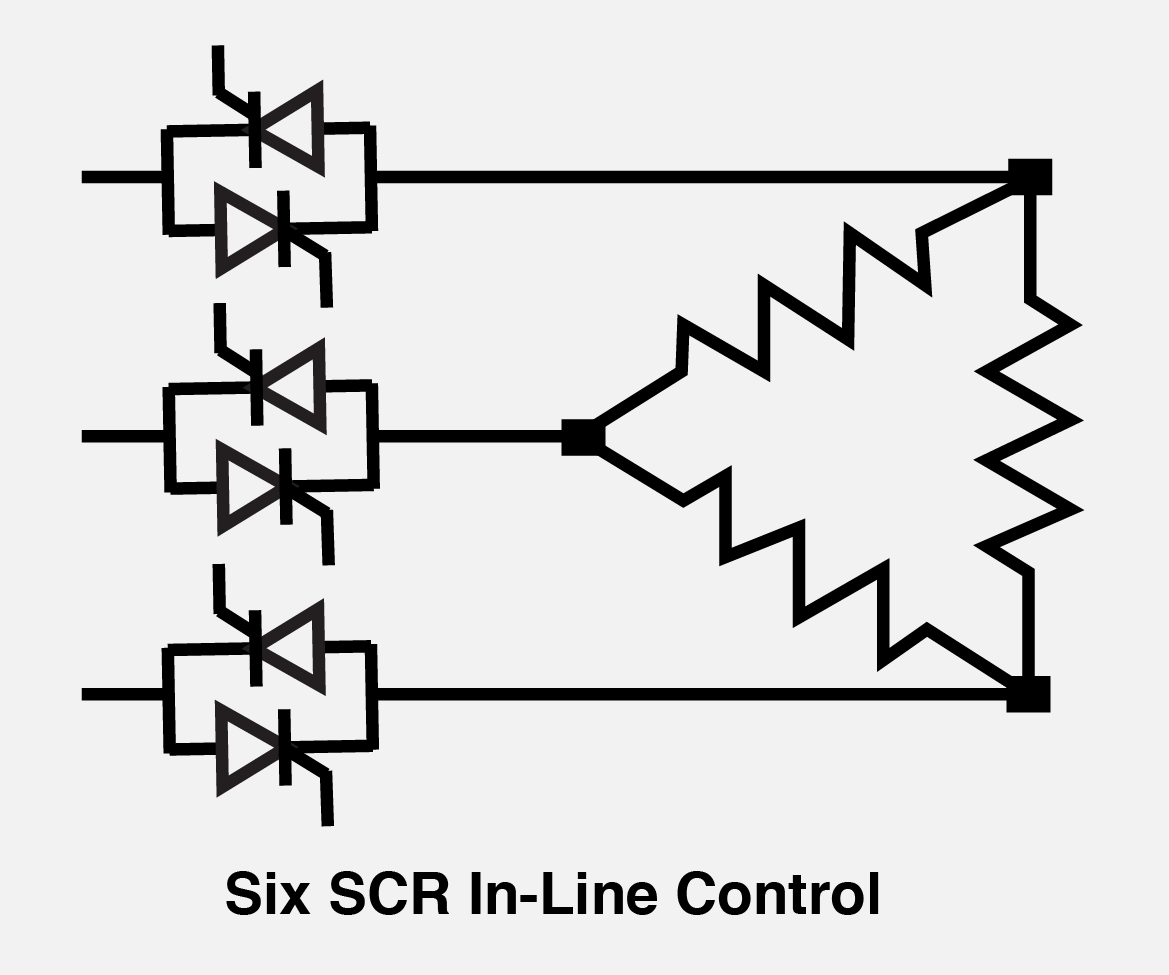ons

Our three-phase controllers are listed here.

#### Phase-Angle Control:

Phase-angle control of three-phase requires a total of 6 power switching devices. These devices can be configured as hybrid (3 SCR's and 3 diodes), 6 SCR in-line or 6 SCR inside delta.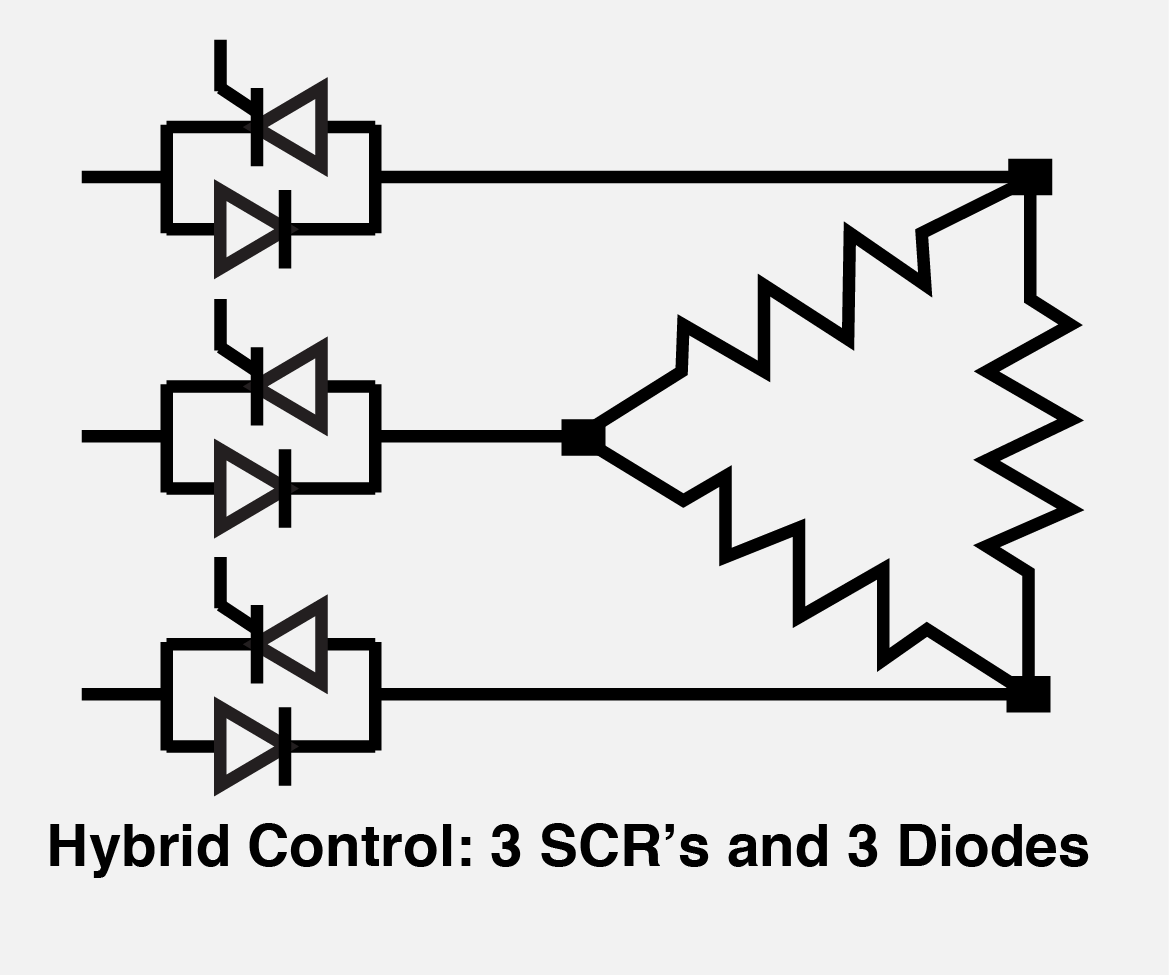#### Hybrid Control:

Three-phase hybrid controllers use three SCR's and three diodes in the power output state. These controllers are intended primarily for three-wire 'wye' or 'delta' type resistive loads connected directly to the controller (i.e., a transformer is not connected between the load and the controller). The three-phase hybrid controller should not be used in a four wire circuit. The fourth wire common return would allow uncontrolled conduction through the power diodes resulting in approximately 50% power output even though the SCR's were off.

The advantage of hybrid controllers is that the cost is somewhat less than six SCR control because the circuit is less complex and diodes are less costly. However, hybrid controllers should not be used if the load is unbalanced. An unbalanced load controlled with a hybrid controller will result in a DC current flow which can result in supply transformer saturation.

####Six SCR in-line controllers:

The Six SCR controller, as the name implies, uses six SCR's to control the load power. This configuration is an ideal configuration for inductive load applications, unbalanced resistive loads and transformer coupled loads.

Caution: if the controller is operating a transformer, either the primary or the secondary must be a delta configuration.

####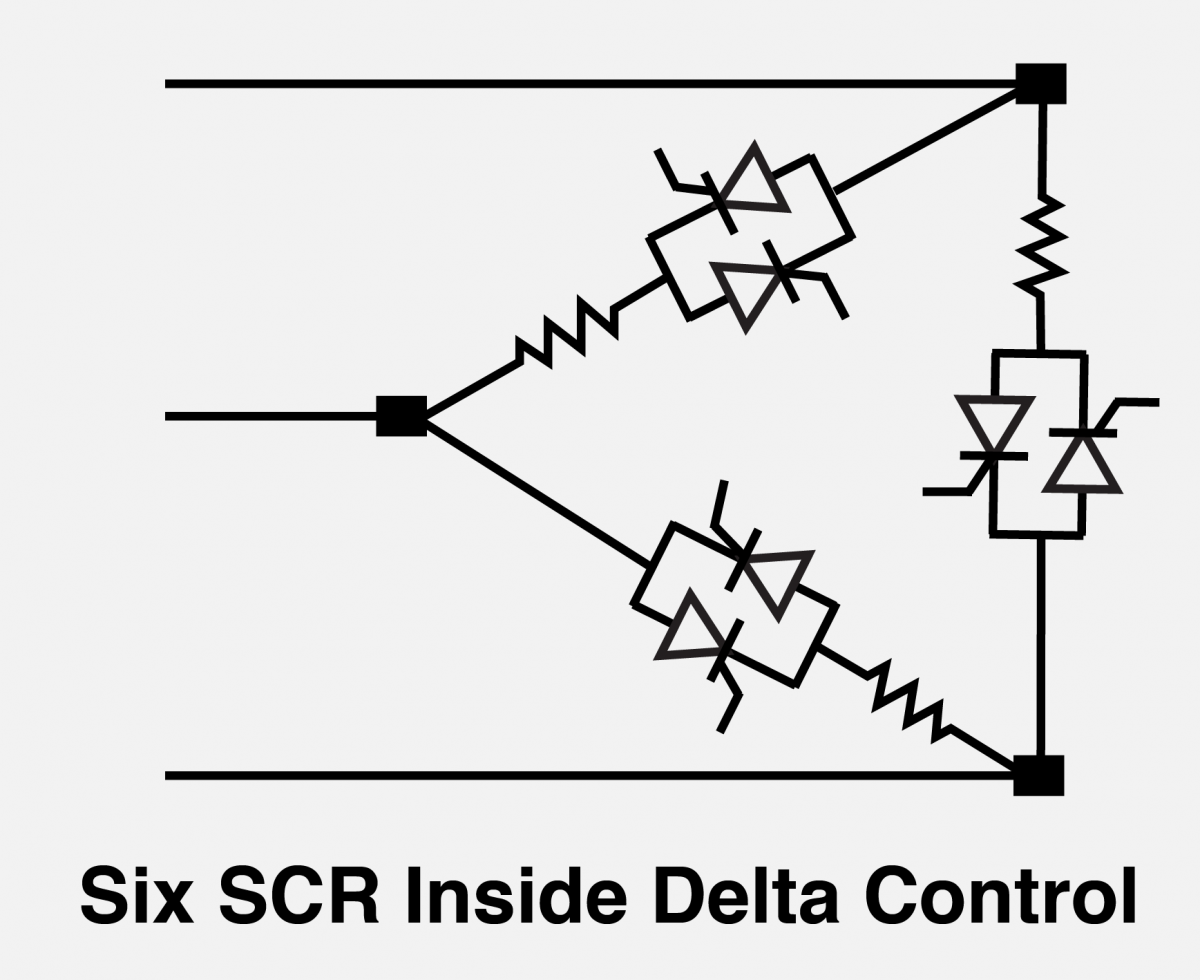Six SCR inside delta control:

Three phase inside delta control is essentially three single-phase controllers operating from the same command or set point. Typically a six SCR controller can be configured to operate inside delta. It is also possible, of course, to configure three single phase controllers controlled by the same command signal. Because the current in each phase is less 57.74% of the line current, smaller and less expensive controllers can be used for inside delta control.

Caution: if inside delta control is used in the primary of a three-phase transformer, a six SCR controller must be used and the secondary must be a delta. (Three single phase controllers cannot be used inside delta to operate a three-phase transformer).

## Zero-Cross Control: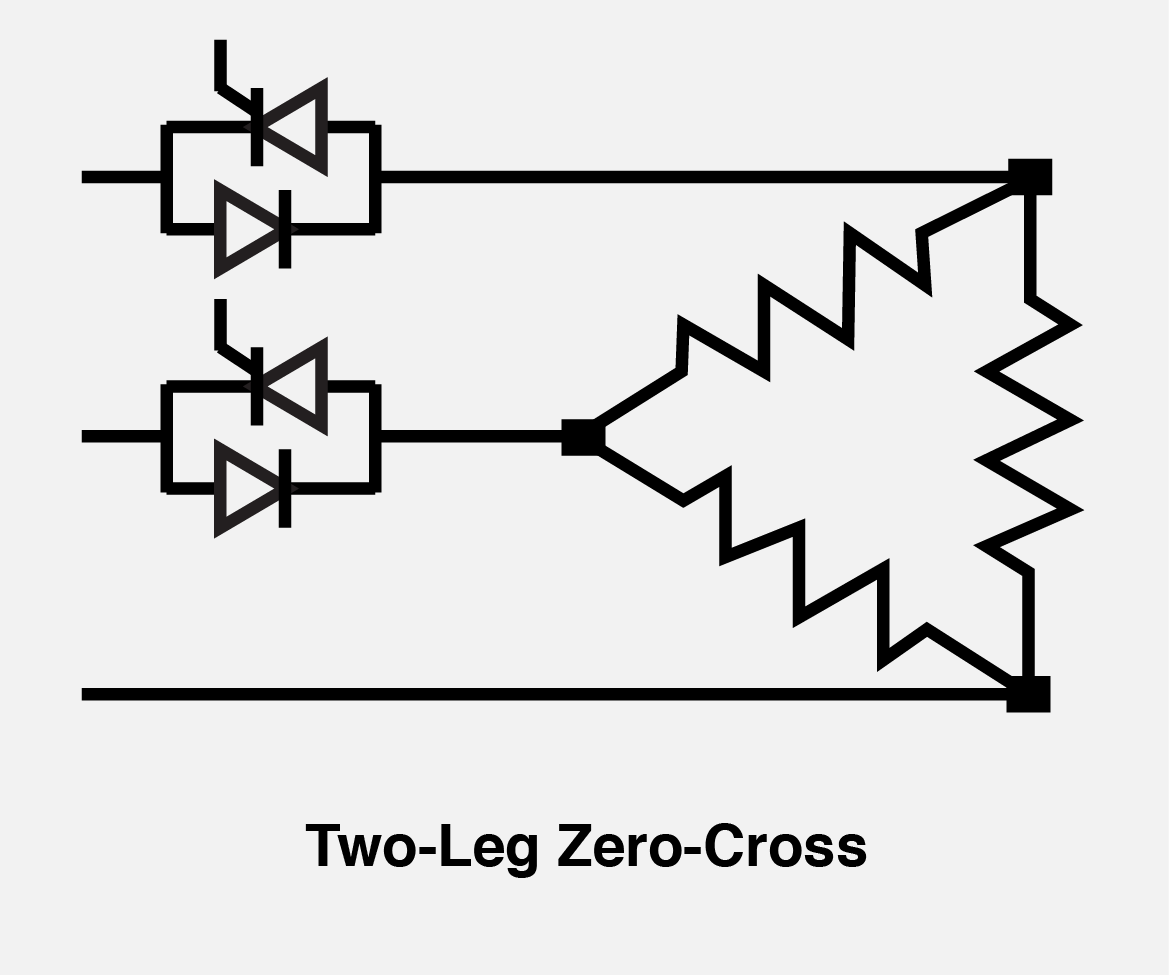#### Two leg control:

This approach uses a pair of back-to-back SCR's in two of the supply leads to the load. If all of the SCR's are off no power can be applied to the load. The two leg control can be used on either 'wye' or 'delta' loads, however, it cannot be used to control four wire loads. The primary advantages of the zero-cross control technique are lower cost and extremely low RFI.

#### Three leg control: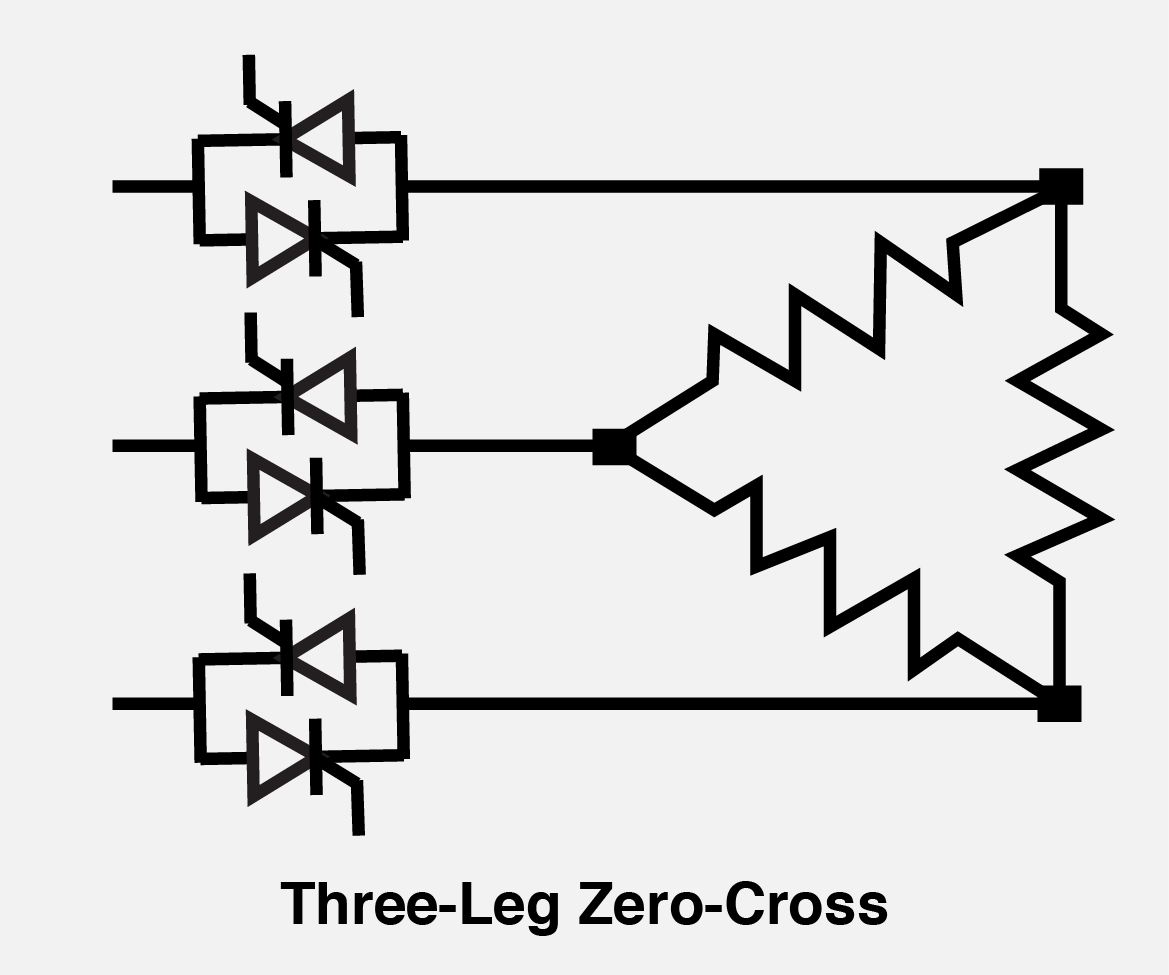Some installations require that all three legs of the load be controlled and therefore back-to-back SCR's are placed in all three supply leads. The cost advantage of zero-cross control is reduced with 3-leg control because of the added SCR's and heat sink costs, however, the circuit cost is less than an equivalent phase-angle controller and the advantage of low RFI is retained.

#### Inside delta:

Zero-cross using the inside delta technique can be used to reduce the current rating of the SCR's, and in some instances, system cost can be reduced.